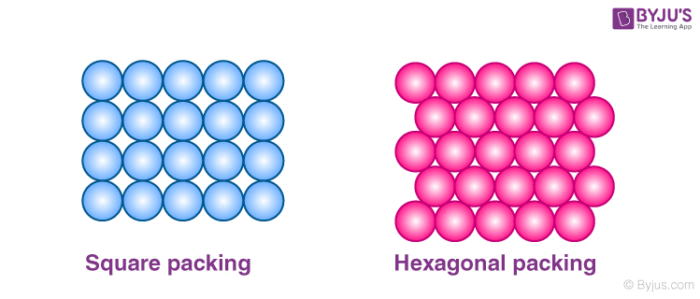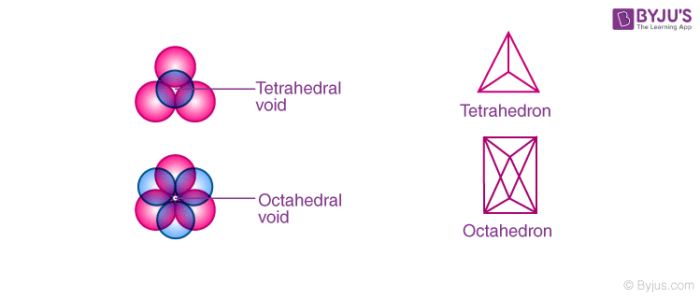# Voids In Solid State

Voids literally mean gaps between constituent particles. Voids in solid states mean the vacant space between the constituent particles in a closed packed structure. Close packing in solids can be generally done in three ways: 1D close packing, 2D close packing and 3D close packing.

In 2 dimensional structures when the atoms are arranged in square close packing and hexagonal close packing, we see empty spaces left over between the atoms. These empty spaces are called voids and in the case of hexagonal packing, these voids are in triangular shapes and are known as the triangular voids.### Packing of Spheres in 2 dimension

These triangular voids in hexagonal packing are shown in two orientations. We can see that in one row, the apex of the triangle points in an upward direction and in the other row it points in a downward direction.

In the three-dimensional structure, both in CCP and HCP close packing in solids, about 26% of total space is vacant and is not covered by spheres. These empty spaces are known as the interstitial voids, interstices or holes. The above voids in solids depend on the number of spheres.

There are two types of interstitial voids in a 3D structure:

1. Tetrahedral voids: In a cubic close packed structure, spheres of the second layer lie above the triangular voids of the first layer. Each sphere touches the three spheres of the first layer. By joining the centre of these four spheres, it forms a tetrahedron and the empty space left over by joining the centre of these spheres forms a tetrahedral void.

In a closed packed structure, the number of tetrahedral voids is two times the number of spheres.

Let the number of spheres be n.

Then the number of tetrahedral voids will be 2n.

1. Octahedral voids: Octahedral voids are found adjacent to the tetrahedral voids. When the triangular voids of the first layer coincide with the triangular voids of the layer above or below it, we get a void that is formed by enclosing six spheres. This vacant space formed by combining the triangular voids of the first layer and that of the second layer is called octahedral voids.

If the number of spheres in a close-packed structure is n, then the number of octahedral voids will be n.Tetrahedral and octahedral void

## Recommended Videos

#### Octahedral Voids#### Tetrahedral Void## Frequently Asked Questions – FAQs

Q1

### What is a tetrahedral void?

A tetrahedral void is basically the empty space that is found in substances having tetrahedral crystal systems. Tetrahedral voids are present among four spheres that have a tetrahedral arrangement.

Q2

### How many octahedral sites are there in BCC?

The six spheres describe regular octahedra, and there is a given space for an interstitial atom in its interior, bordered by six spheres. Octahedral sites occur in crystals such as FCC and BCC.

Q3

### What are octahedral sites?

An octahedral location is a space in the middle of a cluster of six atoms forming an octahedron. The octahedral area is greater than that of the tetrahedral site. Since atoms of the same size are packed together as tightly as possible, each atom has one octahedral site.

Q4

### What are interstitial voids?

Vacuums in solid states mean empty space in a closed packed system between the constituent particles. Such empty spaces are known as the openings, interstices or interstitial voids.

Q5

### Are all solids crystals?

A crystal is a solid where a periodic arrangement of atoms is formed. Not all crystals are solids. For example, as liquid water starts to freeze, the transition in the process begins with tiny ice crystals rising until they merge, creating a polycrystalline structure.

Learning never comes to an end. There is much more to learn in solid states of which voids form just a small part. To know more about the solid states, join BYJU’S classes and feel the difference in the process of learning.

Test Your Knowledge On What Do We Mean By Voids In Solid State!